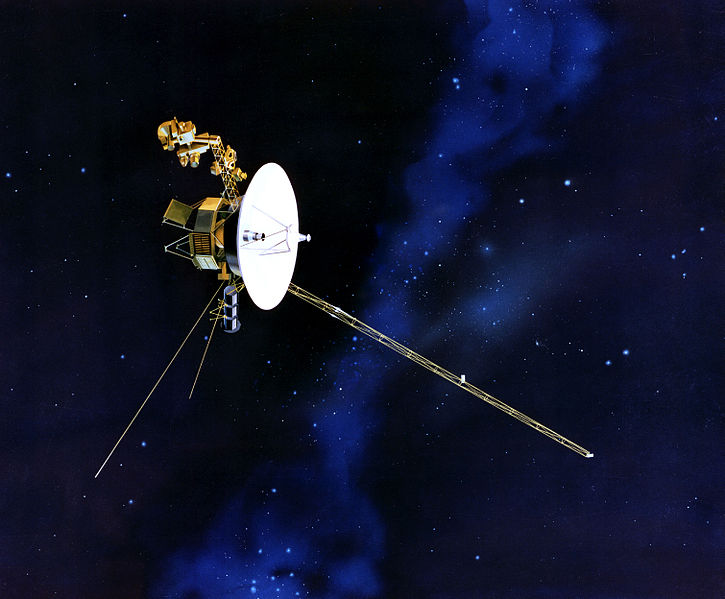# Voyage From The SunAn artist's rendition of Voyager 1 probe

Since the late 1970's, NASA has launched several probes to explore the outer solar system, including Voyagers 1 & 2, Pioneers 10 & 11, and most recently the New Horizons space probe. Sometime in the future, these probes may effectively escape the gravitational influence of the Sun.

If a probe is launched from rest (relative to the Sun) near Earth (which is a distance of $1.50 \times 10^{11} \text{m}$ from the Sun), how much work per unit mass of the probe must be performed on it, if it is to escape the Sun's gravitational pull eventually?

In your calculation, neglect the influence of nearby smaller bodies, such as Earth. Use the fact that the mass of the Sun is $1.99 \times 10^{30} \text{ kg}$ and Newton’s gravitational constant is $6.67 \times 10^{-11} \frac{\text{J} \cdot \text{ m}}{\text{ kg}^2}$. Express your answer in tons of TNT per kilogram; the energy equivalent of 1 ton of TNT is $4.18 \times 10^9 \text{ J}$. Round your answer to 3 significant digits.

Hint: recall that gravitational escape energy is conventionally defined as the work needed to escape to infinity with no excess kinetic energy.

×